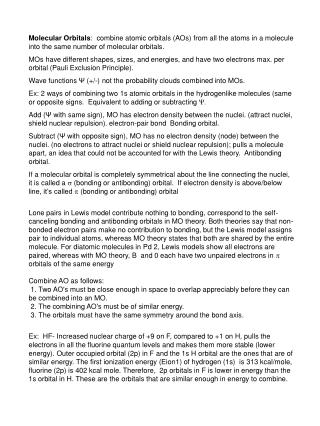DownloadDownload Presentationy = y A - y B

# y = y A - y B

Download Presentation## y = y A - y B

- - - - - - - - - - - - - - - - - - - - - - - - - - - E N D - - - - - - - - - - - - - - - - - - - - - - - - - - -
##### Presentation Transcript

1. Molecular Orbitals: combine atomic orbitals (AOs) from all the atoms in a molecule into the same number of molecular orbitals. MOs have different shapes, sizes, and energies, and have two electrons max. per orbital (Pauli Exclusion Principle). Wave functions Ψ (+/-) not the probability clouds combined into MOs. Ex: 2 ways of combining two 1s atomic orbitals in the hydrogenlike molecules (same or opposite signs. Equivalent to adding or subtracting Ψ. Add (Ψ with same sign), MO has electron density between the nuclei. (attract nuclei, shield nuclear repulsion). electron-pair bond Bonding orbital. Subtract (Ψ with opposite sign),MO has no electron density (node) between the nuclei. (no electrons to attract nuclei or shield nuclear repulsion); pulls a molecule apart, an idea that could not be accounted for with the Lewis theory. Antibonding orbital. If a molecular orbital is completely symmetrical about the line connecting the nuclei, it is called a s (bonding or antibonding) orbital. If electron density is above/below line, it’s called p (bonding or antibonding) orbital Lone pairs in Lewis model contribute nothing to bonding, correspond to the self-canceling bonding and antibonding orbitals in MO theory. Both theories say that non-bonded electron pairs make no contribution to bonding, but the Lewis model assigns pair to individual atoms, whereas MO theory states that both are shared by the entire molecule. For diatomic molecules in Pd 2, Lewis models show all electrons are paired, whereas with MO theory, B and 0 each have two unpaired electrons in p orbitals of the same energy Combine AO as follows:  1. Two AO's must be close enough in space to overlap appreciably before they can be combined into an MO. 2. The combining AO's must be of similar energy. 3. The orbitals must have the same symmetry around the bond axis. Ex: HF- Increased nuclear charge of +9 on F, compared to +1 on H, pulls the electrons in all the fluorine quantum levels and makes them more stable (lower energy). Outer occupied orbital (2p) in F and the 1s H orbital are the ones that are of similar energy. The first ionization energy (Eion1) of hydrogen (1s) is 313 kcal/mole, fluorine (2p) is 402 kcal mole. Therefore, 2p orbitals in F is lower in energy than the 1s orbital in H. These are the orbitals that are similar enough in energy to combine.

2. - + s orbital s orbital resultant y = yA + yB + + + + s2p* s2p* energy energy p2p* p2p* 2p 2p 2p 2p s2p p2p s2p p2p s2s* s2s* 2s 2s 2s 2s s2s s2s MO DIAGRAM: homonuclear diatomic Molecules = Li2 to N2 y = yA - yB MOLECULAR ORBITALS + - energy MO DIAGRAM: homonuclear diatomic Molecules = O2 to F2

3. Fill out the MO diagrams for: 1s 1s 1s 1s He2 H2 s2p* s2p* s2p* p2p* p2p* p2p* 2p 2p 2p 2p 2p 2p s2p s2p p2p s2p p2p p2p s2s* s2s* s2s* 2s 2s 2s 2s 2s 2s s2s s2s s2s Li2 N2 O2 2p 2s p2p* p2p s2p* s2p s2s* s2s F2 2p 2s

4. MOLECULAR ORBITALS: COVALENT AND IONIC BONDING s* EN H = EN Hbonding and antibonding MOs same contribution from both atoms. Pure covalent bond H H H2 nonpolar covalent s s* H EN F > EN HE Bonding MOmore like E F2p AOE antibonding MO more like E H 1s AO form polar covalent bond (partial ionic bond) F HF polar covalent s s* Li EN F >> EN LiE Bonding MO~ E F2p AO Bonding pair essentially given to F, form ionic bond LiF ionic F s